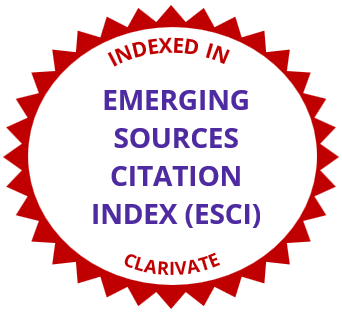# International Electronic Journal of Mathematics EducationAn Integrated Powerpoint-Maple Based Teaching-Learning Model for Multivariate Integral Calculus
• Article Type: Research Article
• International Electronic Journal of Mathematics Education, 2010 - Volume 5 Issue 1, pp. 5-31
https://doi.org/10.29333/iejme/247
• Published Online: 04 Apr 2010
• Article Views: 2444 | Article Download: 3649

# Abstract

In this paper, we present and evaluate a symbolic package based teaching-learning model for triple integral calculus. The model utilizes presentation and visualization technologies to assist in the teaching and learning of mathematical concepts and methods. The key mathematical concepts and integration techniques for triple integrals are introduced to students utilizing the animation feature of PowerPoint display supported by the graphic visualization function of the Maple software. To enhance students’ learning process, a series of workbooks and symbolic tools are developed utilizing Maple 10 to guide students step-by-step in their studies. The developed symbolic tools also enable students to display the three-dimensional (3D) integration domain graphically and to check their calculation results. An investigation undertaken shows that with the proposed teaching-learning model, a higher percentage of students achieved a better understanding of the concepts and a better capacity in evaluating triple integrals with complicated integration domains, compared to traditional teaching and learning with no technology use. We purport that our technology model supports understanding by showing the 3D figures in integration from different angles, and also facilitates independent learning by reducing the need to draw these figures by the students themselves.

# References

• Beltzer, A. I., & Shenkman, A. W. (1995). Use of symbolic computation in engineering education. IEEE Transactions on Education, 38(2), 177–184. doi:10.1109/13.387221
• Buchberger, B. (1989). Should students learn integration rules? ACM SIGSAM Bulletin, 24, 10-17. doi:10.1145/382276.1095228
• Cecil, D. R. (2002). Graphs and enhancing maple multiplication. Mathematics and Computer Education, 36(1), 62–69.
• Cheung, Y. L. (1996). Learning number theory with a computer algebra system. International Journal of Mathematical Education in Science and Technology, 27(3), 379–385.
• Cook, D. (2006). Maple graphing tools for calculus III. Mathematics and Computer Education, 40(1), 36–41.
• Drijvers, P. (2000). Students encountering obstacles using CAS. International Journal of Computers for Mathematical Learning, 5, 189-209.
• Drijvers, P. (2002). Learning mathematics in a computer algebra environment: obstacles are opportunities. ZDM - The International Journal on Mathematics Education, 34(5), 221– 228.
• Fuchs, K. J. (2001). Computer algebra systems in mathematics education. ZDM - The International Journal on Mathematics Education, 35(1), 20–23.
• Hayden, M. B. and Lamagna, E. A. (1998). Newton: An interactive environment for exploring mathematics. Journal of Symbolic Computation, 25, 195–212. doi:10.1006/jsco.1997.0172
• Heid, M. (1988). Resequencing skills and concepts in applied calculus using the computer as a tool. Journal for Research in Mathematics Education, 19(1), 3–25. doi:10.2307/749108
• Johnson, D., & Buege, J. (1995). Rethinking the way we teach undergraduate physics and engineering with Mathematica. In V. Keranen, P. Mitic (Eds.), Mathematics with Vision – Proceedings of the First International Mathematica Symposium (pp.233-242). Southampton, England.
• Kendal, M., & Stacey, K. (2002). Teacher in transition: Moving towards CAS-supported classroom. ZDM - The International Journal on Mathematics Education, 34(5), 196–201.
• Kong, S. C., & Kwok, L. F. (1999). An interactive teaching and learning environment for graph sketching. Computers & Education, 32(1), 1–17. doi:10.1016/S0360- 1315(98)00032-3
• Kramarski, B., & Hirsch, C. (2003). Using computer algebra systems in mathematical classrooms. Journal of Computer Assisted Learning, 19, 35–45. doi:10.1046/j.0266- 4909.2003.00004.x
• Lagrange, J. B. (1999). Complex calculators in the classroom: Theoretical and practical reflections on teaching pre-calculus. International Journal of Computers for Mathematical Learning, 4, 51–81.
• Man, Y. K. (2007). Introducing computer algebra to school teachers of mathematics. Teaching Mathematics and Its Applications, 20(1), 23–26.
• Mathews, J. H. (1990). Using a computer algebra system to teach double integration. International Journal of Mathematical Education in Science and Technology, 21(5), 723– 732. doi: 10.1080/0020739900210506
• Noinang, S., Wiwatanapataphee, B., & Wu, Y.H. (2008). Teaching-learning-tool for integral calculus. Proceedings of the 13th Asian Technology Conference in Mathematics (pp.525- 533). Suan Sunandha Rajabhat University, Bangkok, Thailand.
• Peck, R. (2008). Introduction to statistics and data analysis (3rd ed.). Belmont, CA: Thomson Brooks/Cole.
• Perjési, I. H. (2003). Application of CAS for teaching of integral-transforming theorems. ZDM - The International Journal on Mathematics Education, 35(2). 43–47. doi:10.1007/BF02652771
• Peschek, W., & Schneider, E. (2002). CAS in general mathematics education. ZDM - The International Journal on Mathematics Education, 34(5), 189–195.
• Røyrvik, O. (2002). Teaching electrical engineering using maple. International Journal of Electrical Engineering, 39(4), 297–300.
• Stewart, J. (2003). “Triple integrals-Calculus (5th ed.). Belmont, California: Thomson/ Brooks/ Cole.
• Tall, D. (1991). Recent developments in the use of the computer to visualize and symbolize calculus concepts. MAA Notes, 20, 15–25.
• Tonkes, E. J., Loch, B. I., & Stace, A. W. (2005). An innovation learning model for computation in first year mathematics. International Journal of Mathematical Education in Science and Technology, 36(7), 751–758.
• Ward, J. P. (2003). Modern mathematics for engineers and scientists. Teaching Mathematics and Its Applications, 22(1), 37–44.
• Wu, Y. H., & Wiwatanapataphee, B. (2008). Lecture note: Advanced calculus. Australia: Curtin University of Technology.